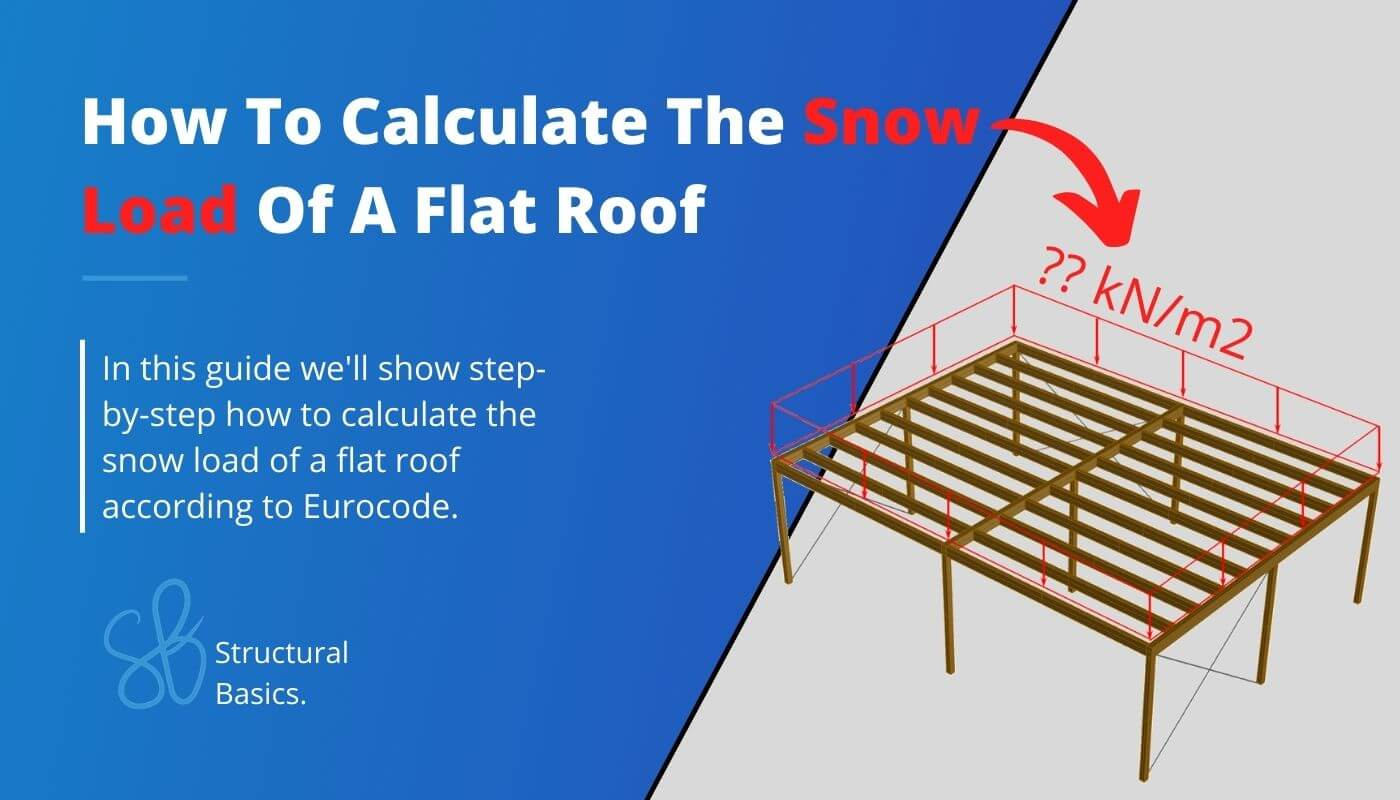# How To Calculate The Snow Load Of A Flat RoofLast updated: June 24th, 2023

Are you building or designing a flat roof, and now you are wondering how to consider the snow load?

Understanding the snow load calculation is crucial to ensure the building structure doesn’t collapse.

So in this post, we’ll show you step-by-step how to calculate the snow load of flat roofs according to Eurocode. 💡💡

Alright, let’s get into it. 🚀🚀

## Example Structure

We’ll calculate the snow load for the flat roof of the below canopy structure.

Examples always help understand the formulas better. 👍👍

## 1. Snow Load on Roofs

For a persistent / tansient design situation EN 1991-1-3 (5.1) is used to calculate the characteristic snow load:

$s = \mu_{i} * C_{e} * C_{t} * s_{k}$

Where

$\mu_{i}$ represents the snow load shape coefficient
$C_{e}$ represents the exposure coefficient
$C_{t}$ represents the thermal coefficient and
$s_{k}$ represents the characteristic snow load value on the ground

Let’s have a closer look how we get those parameters.

## 2. Snow Load Shape Coefficient

The flat roof we are looking at in this example counts as a monopitch roof and therefore the value for $\mu_{1}$ is given in EN 1991-1-3 Table 5.2. For $\alpha$ = 0 which means that the slope of the roof is 0 we get:

$\mu_{1} = 0.8$

## 3. Exposure Coefficient $C_{e}$

EN 1991-1-3 5.2 (7) recommends $C_{e}$ to be taken as 1.0. However, this value depends on the topography of the location. EN 1991-1-3 Table 5.1 categorizes the topography in windswept, normal and sheltered with different values for $C_{e}$. In this blog post, we assume a normal topography for our design. Therefore

$C_{e} = 1.0$

## 4. Thermal Coefficient $C_{t}$

EN 1991-1-3 5.2 (8) defines $C_{t}$ as 1.0. However, this value can be reduced if the roof is covered by glass, which would lead to melting of the snow. In our case, we are not using any glass. Therefore

$C_{t} = 1.0$

## 5. Characteristic Snow Load Value on the Ground $s_{k}$

The characteristic snow load on the ground is found in the national annex of the country your roof is located in. However, there is a great online tool made by Dlubal Software GmbH which calculates the snow load according to location and national annex. Click on the link. Enter the location and the national annex.

If we do that for Copenhagen, Denmark, we get a value of

$s_{k} = 1.0 \frac{kN}{m^2}$

So now we finally have all 4 values required to calculate the characteristic snow load of a flat roof. The snow load for a flat roof located on Copenhagen, Denmark is calculated as

$s = \mu_{i} * C_{e} * C_{t} * s_{k}$
$s = 0.8 * 1.0 * 1.0 * 1.0 \frac{kN}{m^2} = 0.8 \frac{kN}{m^2}$

This load is now applied perpendicular to the roof surface. 👇👇

## Conclusion

Now, that you got an overview of how to calculate the snow load for flat roofs, you can learn about the snow load for pitched roofs and other loads such as:

Because there are always multiple loads acting on structures. Considering these different loads in the structural design is done by setting up Load Combinations with safety factors.🦺

Once all load cases and combinations are set up, the structural elements can be designed. We have already written a guides on how to design structural elements. Check it out!

I hope that this article helped you understand the snow load and how to go further from here. In case you still have questions.

Let us know in the comments below ✍️.

Why is it important to consider the snow load in structural design?

The snow load can lead to significant forces and stresses of a building’s structure. If it’s not accounted for in the design, it can lead to structural failure or collapse.

How is the snow load calculated according to Eurocode?

Eurocode provides formulas and coefficients for calculating the snow load based on factors such as the location of the building, the type of roof, the slope of the roof and many more.
These formulas and coefficients can vary from country to country because every country has its own National Annex.

How is the type of roof taken into account in the snow load calculations?

The shape and pitch of the roof can affect how snow accumulates on the surface. Eurocode provides different coefficients for different types of roofs (flat roofs, pitched roofs, arches, roofs with a parapet, etc.).